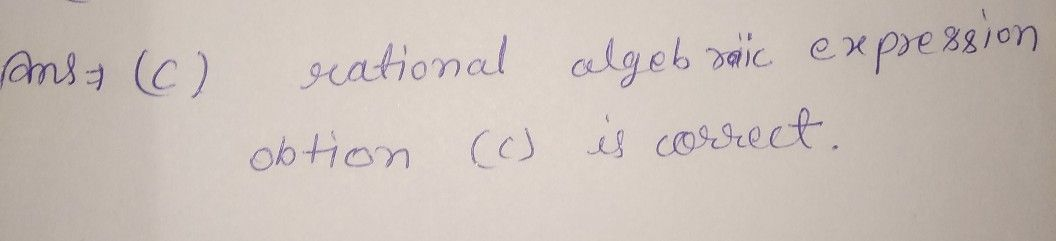Symbol
Problem$10$ Which of the following terms is described as "the ratio of two polynomial expressions"? A. linear algebraic expression B. linear algebraic equation C. rational algebraic expression D. rational algebraic equation
7th-9th grade
Calculus
Search count: 116
SolutionQanda teacher - vikaskanti